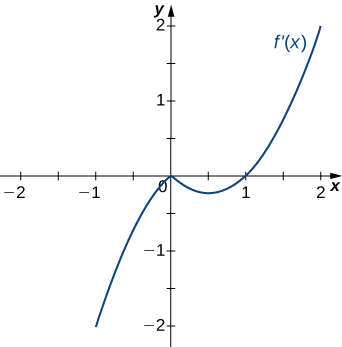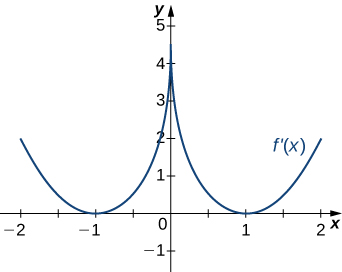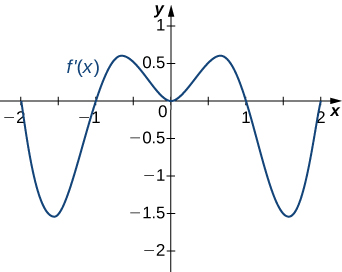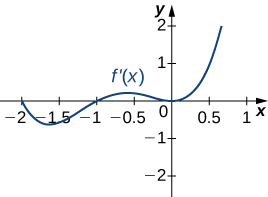$$\newcommand{\id}{\mathrm{id}}$$ $$\newcommand{\Span}{\mathrm{span}}$$ $$\newcommand{\kernel}{\mathrm{null}\,}$$ $$\newcommand{\range}{\mathrm{range}\,}$$ $$\newcommand{\RealPart}{\mathrm{Re}}$$ $$\newcommand{\ImaginaryPart}{\mathrm{Im}}$$ $$\newcommand{\Argument}{\mathrm{Arg}}$$ $$\newcommand{\norm}{\| #1 \|}$$ $$\newcommand{\inner}{\langle #1, #2 \rangle}$$ $$\newcommand{\Span}{\mathrm{span}}$$

# 3.5E: Exercises

$$\newcommand{\vecs}{\overset { \rightharpoonup} {\mathbf{#1}} }$$ $$\newcommand{\vecd}{\overset{-\!-\!\rightharpoonup}{\vphantom{a}\smash {#1}}}$$$$\newcommand{\id}{\mathrm{id}}$$ $$\newcommand{\Span}{\mathrm{span}}$$ $$\newcommand{\kernel}{\mathrm{null}\,}$$ $$\newcommand{\range}{\mathrm{range}\,}$$ $$\newcommand{\RealPart}{\mathrm{Re}}$$ $$\newcommand{\ImaginaryPart}{\mathrm{Im}}$$ $$\newcommand{\Argument}{\mathrm{Arg}}$$ $$\newcommand{\norm}{\| #1 \|}$$ $$\newcommand{\inner}{\langle #1, #2 \rangle}$$ $$\newcommand{\Span}{\mathrm{span}}$$ $$\newcommand{\id}{\mathrm{id}}$$ $$\newcommand{\Span}{\mathrm{span}}$$ $$\newcommand{\kernel}{\mathrm{null}\,}$$ $$\newcommand{\range}{\mathrm{range}\,}$$ $$\newcommand{\RealPart}{\mathrm{Re}}$$ $$\newcommand{\ImaginaryPart}{\mathrm{Im}}$$ $$\newcommand{\Argument}{\mathrm{Arg}}$$ $$\newcommand{\norm}{\| #1 \|}$$ $$\newcommand{\inner}{\langle #1, #2 \rangle}$$ $$\newcommand{\Span}{\mathrm{span}}$$

### Exercise $$\PageIndex{1}$$

1) If c is a critical point of $$f(x)$$, when is there no local maximum or minimum at $$c$$? Explain.

2) For the function $$y=x^3$$, is $$x=0$$ both an inflection point and a local maximum/minimum?

It is not a local maximum/minimum because $$f′$$ does not change sign

3) For the function $$y=x^3$$, is $$x=0$$ an inflection point?

4) Is it possible for a point $$c$$ to be both an inflection point and a local extrema of a twice differentiable function?

No

6) Why do you need continuity for the first derivative test? Come up with an example.

7) Explain whether a concave-down function has to cross $$y=0$$ for some value of $$x$$.

False; for example, $$y=\sqrt{x}$$.

8) Explain whether a polynomial of degree $$2$$ can have an inflection point.

Under Construction.

### Exercise $$\PageIndex{2}$$

For the following exercises, analyze the graphs of $$f′$$, then list all intervals where f is increasing or decreasing.

1)Increasing for $$−2<x<−1$$ and $$x>2$$; decreasing for $$x<−2$$ and $$−1<x<2$$

2)3)Decreasing for $$x<1$$, increasing for $$x>1$$

4)5)Decreasing for $$−2<x<−1$$ and $$1<x<2$$; increasing for $$−1<x<1$$ and $$x<−2$$ and $$x>2$$

### Exercise $$\PageIndex{3}$$

For the following exercises, analyze the graphs of $$f′,$$ then list all intervals where

a. $$f$$ is increasing and decreasing and

b. the minima and maxima are located.1)a. Increasing over $$−2<x<−1,0<x<1,x>2$$, decreasing over $$x<−2, −1<x<0,1<x<2;$$ b. maxima at $$x=−1$$ and $$x=1$$, minima at $$x=−2$$ and $$x=0$$ and $$x=2$$

2)3)a. Increasing over $$x>0$$, decreasing over $$x<0;$$ b. Minimum at $$x=0$$

4)### Exercise $$\PageIndex{4}$$

For the following exercises, analyze the graphs of $$f′$$, then list all inflection points and intervals $$f$$ that are concave up and concave down.

1)Concave up on all $$x$$, no inflection points

2)3)Concave up on all $$x$$, no inflection points

4)5)Concave up for $$x<0$$ and $$x>1$$, concave down for $$0<x<1$$, inflection points at $$x=0$$ and $$x=1$$

### Exercise $$\PageIndex{5}$$

For the following exercises, draw a graph that satisfies the given specifications for the domain $$x=[−3,3].$$ The function does not have to be continuous or differentiable.

1) $$f(x)>0,f′(x)>0$$ over $$x>1,−3<x<0,f′(x)=0$$ over $$0<x<1$$

2) $$f′(x)>0$$ over $$x>2,−3<x<−1,f′(x)<0$$ over $$−1<x<2,f''(x)<0$$ for all $$x$$

3) $$f''(x)<0$$ over $$−1<x<1,f''(x)>0,−3<x<−1,1<x<3,$$ local maximum at $$x=0,$$ local minima at $$x=±2$$

4) There is a local maximum at $$x=2,$$ local minimum at $$x=1,$$ and the graph is neither concave up nor concave down.

5) There are local maxima at $$x=±1,$$ the function is concave up for all $$x$$, and the function remains positive for all $$x.$$

Under Construction

### Exercise $$\PageIndex{6}$$

For the following exercises, determine

a. intervals where $$f$$ is increasing or decreasing and

b. local minima and maxima of $$f$$.

1) $$f(x)=sinx+sin^3x$$ over −π<x<π

a. Increasing over $$−\frac{π}{2}<x<\frac{π}{2},$$ decreasing over $$x<−π\frac{π}{2},x>\frac{π}{2}$$

b. Local maximum at $$x=\frac{π}{2}$$; local minimum at $$x=−\frac{π}{2}$$

2) $$f(x)=x^2+cosx$$

Under Construction

### Exercise $$\PageIndex{7}$$

For the following exercises, determine

a. intervals where $$f$$ is increasing or decreasing,

b. local minima and maxima of $$f$$,

c. intervals where $$f$$ is concave up and concave down, and

d. the inflection points of $$f.$$

1) $$f(x)=x^3−4x^2+x+2$$

a. Concave up for $$x>\frac{4}{3},$$ concave down for $$x<\frac{34}{3}$$

b. Inflection point at $$x=\frac{4}{3}$$

2) $$f(x)=x^2−6x$$

3) $$f(x)=x^3−6x^2$$

a. Increasing over $$x<0$$ and $$x>4,$$ decreasing over $$0<x<4$$

b. Maximum at $$x=0$$, minimum at $$x=4$$

c. Concave up for $$x>2$$, concave down for $$x<2$$

d. Infection point at $$x=2$$

4) $$f(x)=x^4−6x^3$$

5) $$f(x)=x^{11}−6x^{10}$$

a. Increasing over $$x<0$$ and $$x>\frac{60}{11}$$, decreasing over $$0<x<\frac{60}{11}$$

b. Minimum at $$x=\frac{60}{11}$$

c. Concave down for $$x<\frac{54}{11}$$, concave up for $$x>\frac{54}{11}$$

d. Inflection point at $$x=\frac{54}{11}$$

6) $$f(x)=x+x^2−x^3$$

7) $$f(x)=x^2+x+1$$

a. Increasing over $$x>−\frac{1}{2}$$, decreasing over $$x<−\frac{1}{2}$$

b. Minimum at $$x=−\frac{1}{2}$$

c. Concave up for all $$x$$

d. No inflection points

8) $$f(x)=x^3+x^4$$

### Exercise $$\PageIndex{8}$$

For the following exercises, determine

a. intervals where $$f$$ is increasing or decreasing,

b. local minima and maxima of $$f,$$

c. intervals where $$f$$ is concave up and concave down, and

d. the inflection points of $$f.$$ Sketch the curve, then use a calculator to compare your answer. If you cannot determine the exact answer analytically, use a calculator.

1) $$f(x)=sin(πx)−cos(πx)$$ over $$x=[−1,1]$$

a. Increases over $$−\frac{1}{4}<x<\frac{3}{4},$$ decreases over $$x>\frac{3}{4}$$ and $$x<−\frac{1}{4}$$

b. Minimum at $$x=−\frac{1}{4}$$, maximum at $$x=\frac{3}{4}$$

c. Concave up for $$−\frac{3}{4}<x<\frac{1}{4}$$, concave down for $$x<−\frac{3}{4}$$ and $$x>\frac{1}{4}$$

d. Inflection points at $$x=−\frac{3}{4},x=\frac{1}{4}$$

2) $$f(x)=x+sin(2x)$$ over $$x=[−\frac{π}{2},\frac{π}{2}]$$

3) $$f(x)=sinx+tanx$$ over $$(−\frac{π}{2},\frac{π}{2})$$

a. Increasing for all $$x$$

b. No local minimum or maximum

c. Concave up for $$x>0$$, concave down for $$x<0$$

d. Inflection point at $$x=0$$

4) $$f(x)=(x−2)^2(x−4)^2$$

5) $$f(x)=\frac{1}{1−x},x≠1$$

a. Increasing for all $$x$$ where defined

b. No local minima or maxima

c. Concave up for $$x<1$$; concave down for $$x>1$$

d. No inflection points in domain

6) $$f(x)=\frac{sinx}{x}$$ over $$x=[−2π,2π] [2π,0)∪(0,2π]$$

7) $$f(x)=sin(x)e^x$$ over $$x=[−π,π]$$

a. Increasing over $$−\frac{π}{4}<x<\frac{3π}{4}$$, decreasing over $$x>\frac{3π}{4},x<−\frac{π}{4}$$

b. Minimum at $$x=−\frac{π}{4}$$, maximum at $$x=\frac{3π}{4}$$

c. Concave up for $$−\frac{π}{2}<x<\frac{π}{2}$$, concave down for $$x<−\frac{π}{2},x>\frac{π}{2}$$

d. Infection points at $$x=±\frac{π}{2}$$

8) $$f(x)=lnx\sqrt{x},x>0$$

9) $$f(x)=\frac{1}{4}\sqrt{x}+\frac{1}{x},x>0$$

a. Increasing over $$x>4,$$ decreasing over $$0<x<4$$

b. Minimum at $$x=4$$

c. Concave up for $$0<x<8\sqrt{2}$$, concave down for $$x>8\sqrt{2}$$

d. Inflection point at $$x=8\sqrt{2}$$

10) $$f(x)=\frac{e^x}{x},x≠0$$

11) $$f(x)=\frac{x}{ln(|x|)}$$

a. Increasing over $$x>e,$$ decreasing over $$-1<x<e$$

b. Minimum at $$x=e$$

c. Concave up for $$(-1,0) \cup (1, \infty)\$$, concave down for $$(0,1)$$

d. Inflection point at $$x=0$$

### Exercise $$\PageIndex{9}$$

For the following exercises, interpret the sentences in terms of $$f,f′,$$ and $$f''.$$

1) The population is growing more slowly. Here $$f$$ is the population.

$$f>0,f′>0,f''<0$$

2) A bike accelerates faster, but a car goes faster. Here $$f=$$ Bike’s position minus Car’s position.

3) The airplane lands smoothly. Here $$f$$ is the plane’s altitude.

$$f>0,f′<0,f''<0$$

4) Stock prices are at their peak. Here $$f$$is the stock price.

5) The economy is picking up speed. Here $$f$$ is a measure of the economy, such as GDP.

$$f>0,f′>0,f''>0$$

### Exercise $$\PageIndex{10}$$

For the following exercises, consider a third-degree polynomial $$f(x),$$ which has the properties f′(1)=0,f′(3)=0.

Determine whether the following statements are true or false. Justify your answer.

1) $$f(x)=0$$ for some $$1≤x≤3$$

2) $$f''(x)=0$$ for some $$1≤x≤3$$

True, by the Mean Value Theorem

3) There is no absolute maximum at $$x=3$$

4) If $$f(x)$$ has three roots, then it has $$1$$ inflection point.

True, examine derivative

5) If $$f(x)$$ has one inflection point, then it has three real roots.

\begin{enumerate}

\item {Consider the function $$f(x)=x^2 e^{-x}.$$

\begin{enumerate}

\item Find the interval(s) on which $f(x)$ is increasing and

\item Find the interval(s) on which $f(x)$ is decreasing and

\item Find the local (relative) maximum and local (relative) minimum (if any).

%\vspace{8in}

\end{enumerate}}

\item {Consider the function $$f(x)=3(x^2-4)^{2/3}.$$

\begin{enumerate}

\item Find the interval(s) on which $f(x)$ is increasing and

\item Find the interval(s) on which $f(x)$ is decreasing and

\item Find the local (relative) maximum and local (relative) minimum (if any).

%\vspace{8in}

\end{enumerate}}

\item Consider the function $$g(x)=10x^3+ 3x^5.$$ Find

\begin{enumerate}

\item the interval(s) on which $g(x)$ is concave up and

\item the interval(s) on which $g(x)$ is concave down and

\item inflection points ( if any).

\end{enumerate}

\item Consider the function $$g(x)=e^{-x^2/2}.$$ Find

\begin{enumerate}

\item the interval(s) on which $g(x)$ is concave up and

\item the interval(s) on which $g(x)$ is concave down and

\item inflection points ( if any).

\end{enumerate}

\item{ Find horizontal asymptote of the function $$y=f(x)= x- \sqrt{x^2+2x-6}.$$}

%\vspace{8 in}

\item { Consider the function $$f(x)=x e^{-2x}.$$

\begin{enumerate}

\item Find the interval(s) on which $f(x)$ is increasing and

\item Find the interval(s) on which $f(x)$ is decreasing and

\item Find the local (relative) maximum and local (relative) minimum (if any).

%\vspace{8in}

\end{enumerate}}

\item { Consider the function $$f(x)=x^2 e^{-x}.$$

\begin{enumerate}

\item Find the interval(s) on which $f(x)$ is increasing and

\item Find the interval(s) on which $f(x)$ is decreasing and

\item Find the local (relative) maximum and local (relative) minimum (if any).

%\vspace{8in}

\end{enumerate}}

\item { Consider the function $$f(x)=x+\frac{1}{x}.$$

\begin{enumerate}

\item Find the interval(s) on which $f(x)$ is increasing and

\item Find the interval(s) on which $f(x)$ is decreasing and

\item Find the local (relative) maximum and local (relative) minimum (if any).

%\vspace{8in}

\end{enumerate}}

\item { Consider the function $$g(x)=5x^3+ 2x^5.$$ Find

\begin{enumerate}

\item the interval(s) on which $g(x)$ is concave up and

\item the interval(s) on which $g(x)$ is concave down and

\item inflection points ( if any).

%\vspace{8in}

\end{enumerate}}

\item { Consider the function $$g(x)=\ln(1+x^2).$$ Find

\begin{enumerate}

\item the interval(s) on which $g(x)$ is concave up and

\item the interval(s) on which $g(x)$ is concave down and

\item inflection points ( if any).

%\vspace{8in}

\end{enumerate}}

\item { Consider the function $$g(x)=(3-x^2)^2.$$ Find

\begin{enumerate}

\item the interval(s) on which $g(x)$ is concave up and

\item the interval(s) on which $g(x)$ is concave down and

\item inflection points ( if any).

%\vspace{8in}

\end{enumerate}}

\item { Consider the function

$$\displaystyle{f(t)=\frac{1}{1-t^2}}$$

b) Find vertical and horizontal asymptotes (if any).

c) Find the intervals when $f(t)$ is concave up and concave down.

}

\item {Consider the function

$$\displaystyle{f(t)=e^{-t^2}}$$

a) Find the local maxima and minima for the function $f(t)$.

b) Find the intervals when $f(t)$ is increasing and decreasing.

c) Find the intervals when $f(t)$ is concave up and down.

d) Find the inflection points for $f(t)$.

}

\item { Find all vertical and

horizontal asymptotes for the function

$$\displaystyle{f(x)=\frac{x^2-5x+6}{x^2-4x+3}}$$

}

\item Suppose we have the following

information, where

$$y=\frac{x}{x^2-1},\qquad y^\prime=-\frac{x^2+1}{\left(x^2-1\right)^2}, \mbox{ and }y^{\prime\prime}=\frac{2x\left(x^2+3\right)}{\left(x^2-1 \right)^3}.$$

\noindent a) Identify any asymptotes for the function $y$ using limits.

\noindent b) Find where the function $y$ is

increasing/decreasing and any relative extrema.

\noindent c) Find where the function $y$ is concave

up/down and any points of inflection.

\noindent d) Graph the function $y$.

\item Let $f(x) = x^{5/4}-x^{1/4}.$ Determine the intervals on which $f$ is increasing and decreasing and find any local extrema.

\item Let $g(x) = \ln (x^2+1).$ Determine the intervals of concavity of $g(x)$ and locate any inflection points. \item Let $f(x) = x^2e^{-16x^2}.$ Determine the intervals on which $f$ is increasing and decreasing and find any local extrema.

\item Let $g(x) = (\ln x)^2.$ Determine the intervals of concavity of $g(x)$ and locate any inflection points. \item Given a function $\displaystyle{y=f(x)= x-2tan^{-1}(x)}$, find interval(s) on which the function is concave

up and the interval(s) on which the function is concave down, and inflection point(s).

\item Let $g(x) = x-2 \tan^{-1}(x).$ Determine the intervals of concavity of $g(x)$ and locate any inflection points.

\end{enumerate}